# Apply Alternate Segment Theorem

In this worksheet, students will learn the alternate segment theorem and be able to apply it.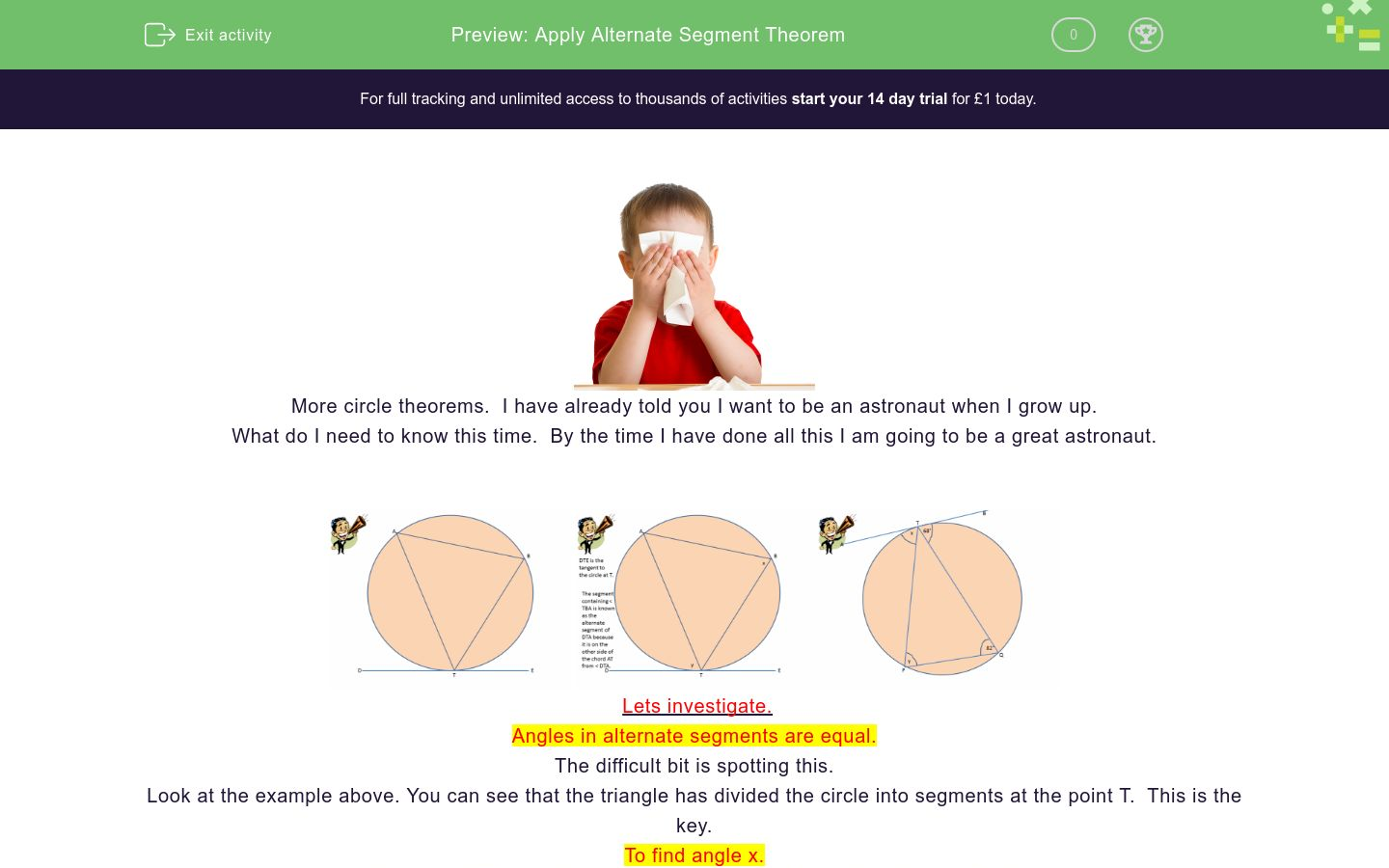Key stage:  KS 4

GCSE Subjects:   Maths

GCSE Boards:   OCR, AQA, Eduqas, Pearson Edexcel

Curriculum topic:   Basic Geometry, Geometry and Measures

Curriculum subtopic:   Circles, Properties and Constructions

Difficulty level:### QUESTION 1 of 10More circle theorems.  I have already told you I want to be an astronaut when I grow up.

What do I need to know this time.  By the time I have done all this I am going to be a great astronaut.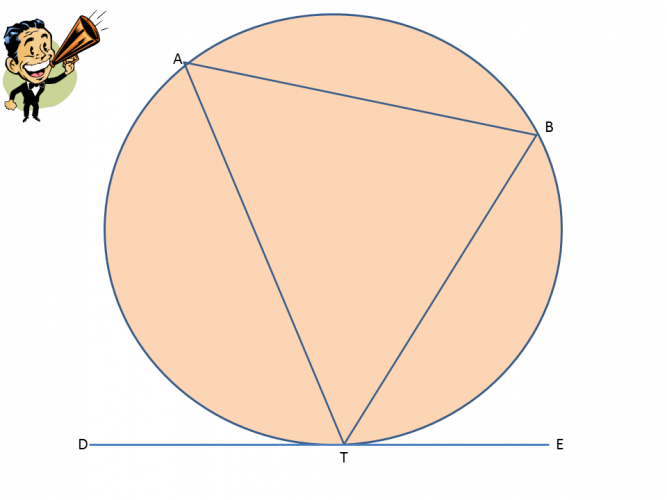Lets investigate.

Angles in alternate segments are equal.

The difficult bit is spotting this.

Look at the example above. You can see that the triangle has divided the circle into segments at the point T.  This is the key.

To find angle x.

Angle x is ATP is in the alternate segment to 82°, so therefore x is 82°.

To find angle y.

Angle y is QPT is in the opposite segment to 68°, so therefore y is also 68°.

As with all circle theorems you will need to recall basic angle properties, so watch out for these.There is only one way to tackle these.  Just jump in.  it is good training for astronauts at least.66° 76° 86° Angle x is Angle y is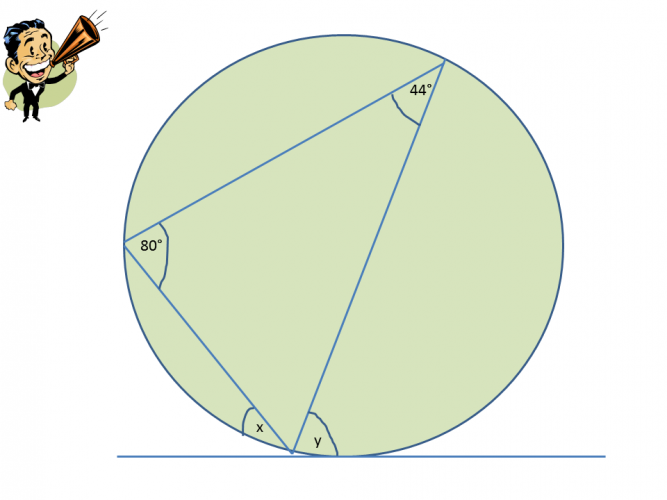66° 76° 86° Angle x is Angle y is## Column B

Angle x =
52°
Angle y =
47°
Angle z =
81°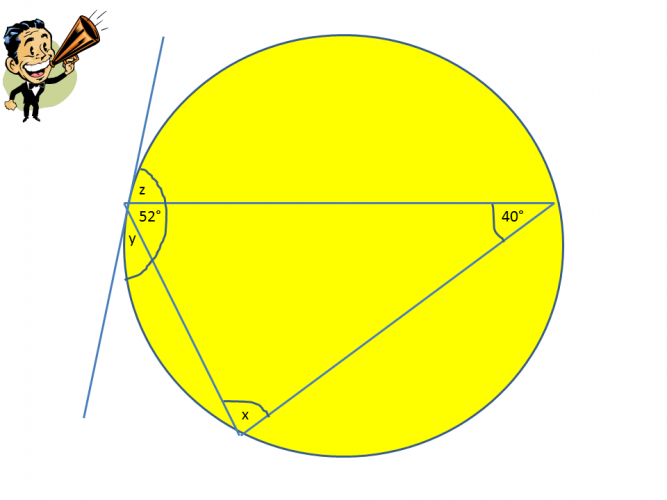Angle x = 40° 52° 88° Angle y = 40°, 52°, 88° Angle z = 40° 52° 88°Angle x = 40° 52° 88° Angle y = 40°, 52°, 88° Angle z = 40° 52° 88°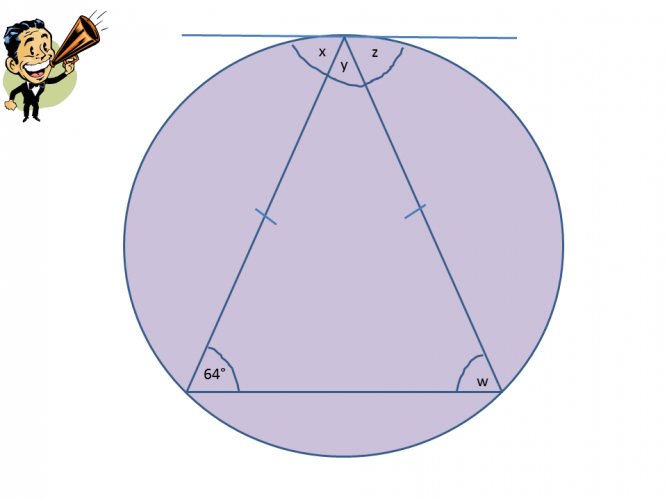52° 64° 82° Angle w Angle x Angle y Angle zWhat is the value of y.Angle x and y are in alternate segments so their value is the same.

What is the value.100°

80°

50°

70°36° 108° 72° 98° The value of x is
• Question 166° 76° 86° Angle x is Angle y is
EDDIE SAYS
Did you spot this okay? Go to one given angle and look for the letter in the alternate segment. Also you can see that y is in the same segment as 76° so it can't be that one.
• Question 2EDDIE SAYS
Angle x is opposite to 44° so therefore in the alternate segment. Similarly angle y is opposite to 80° and is in its alternate segment. We know that angles in alternate segments are the same.
• Question 3## Column B

Angle x =
52°
Angle y =
81°
Angle z =
47°
EDDIE SAYS
Hopefully you are looking for the opposite angle to the one given. Opposites attract after all (or so they say). This should have given you x = 52° and y = 81°. You now have two ways to work out angle Z. Either angles in a triangle add up to 180° 180 - 52 - 81 = 47° or angles on a straight line add up to 180° 180 - 52 - 81 = 47° Its great when there are different options.
• Question 4Angle x = 40° 52° 88° Angle y = 40°, 52°, 88° Angle z = 40° 52° 88°
EDDIE SAYS
You have to be careful on questions like this they can prove a little tricky. You have two angles in the triangle already so work out angle x first. 180 - 52 - 40 = 88°. Angle y is in the alternative segment to 40° leaving angle z in the alternative segment to x.
• Question 5EDDIE SAYS
Find angle x first using the rule of angles on a straight line add up to 180° i.e 180 - 63 - 38 = 79° Now you should see that angle y is in the alternate segment to 63° and angle z is in the alternate segment to x. Can you see where learning basic angle properties can make our higher level maths easier?
• Question 652° 64° 82° Angle w Angle x Angle y Angle z
EDDIE SAYS
Are you spotting the alternate segments now and knowing when to apply another rule? The help of an isosceles triangle make things a little easier as the two base angles are the same, hence angle w 64° Angles x and z are in the alternate segments to the base angles, leaving just angle y to work out using angles on a straight line. 180 - 64 - 64 = 52°
• Question 7What is the value of y.

84
EDDIE SAYS
Here you will need to find the value of x first. Use the markings on the triangle to see that it is isosceles meaning angle w is also 48°. From here you can find x 180 - 48 - 48 = 84° x is in the alternate segment to y, hence y is 84°
• Question 8Angle x and y are in alternate segments so their value is the same.

What is the value.

52
EDDIE SAYS
Did you find this tricky? First calculate the missing angle on the straight line with 104°. 180 - 104 = 76° The tangents that hit the edge of the circle make the triangle isosceles. To find angle x 180 - 76 = 104 ÷ 2 = 52° Y is in the alternate segment so also 52°
• Question 950°
EDDIE SAYS
The triangle with the angle in is isosceles making the base angles 50° each. x is in the alternate segment so therefore also 50°
• Question 1036° 108° 72° 98° The value of x is
EDDIE SAYS
What no numbers...... No worries. We have an isosceles triangle so the base angles are the same. We are given no other information so have to assume the base angles have a value of x. There would be 5x in the triangle so 5x = 180. 180 ÷ 5 = 36° x is in the alternate segment to the base angle so therefore also 36°.
---- OR ----

Sign up for a £1 trial so you can track and measure your child's progress on this activity.

### What is EdPlace?

We're your National Curriculum aligned online education content provider helping each child succeed in English, maths and science from year 1 to GCSE. With an EdPlace account you’ll be able to track and measure progress, helping each child achieve their best. We build confidence and attainment by personalising each child’s learning at a level that suits them.

Get started